Solids built with 12 decagons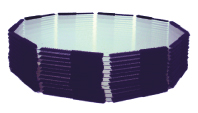Build a truncated dodecahedron by joining two decagons and one triangle in each vertex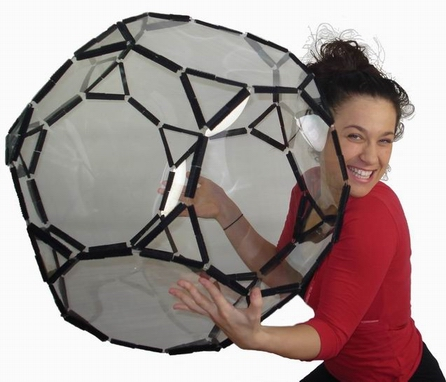Triangles sold separately Build a rhombicosidodecahedron by joining one decagon, one hexagon and one square in each vertex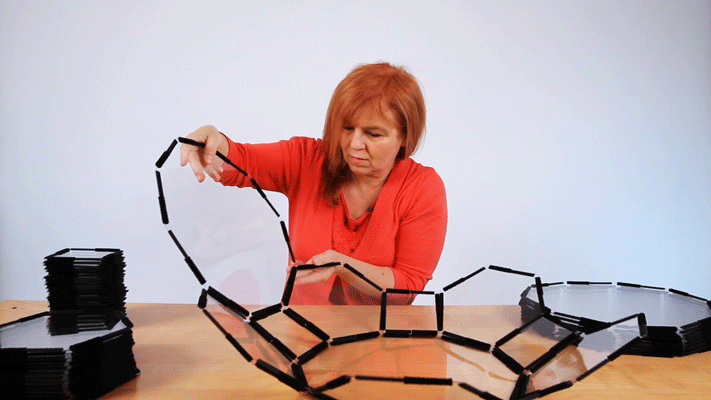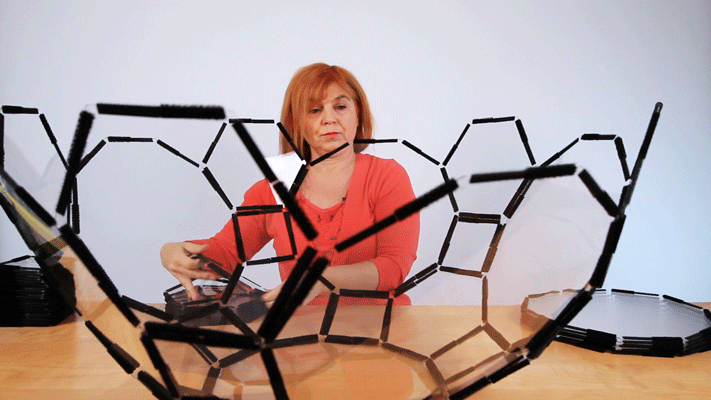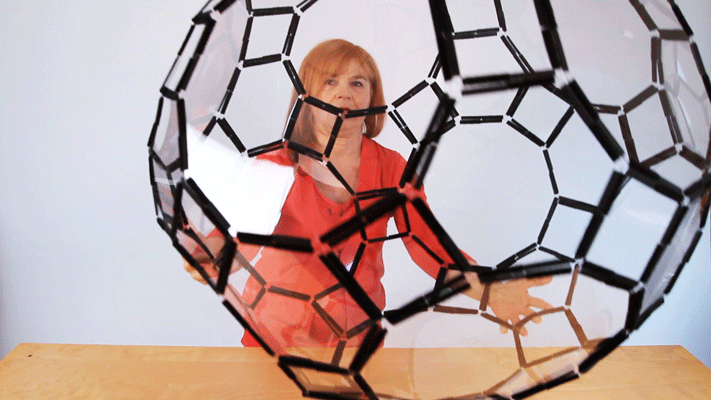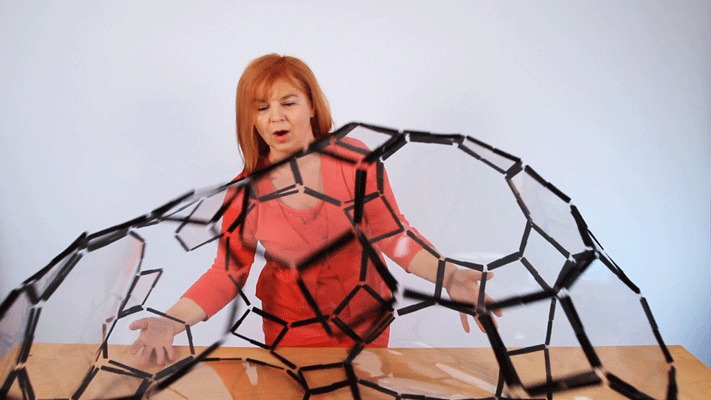Hexagons and squares sold separately